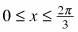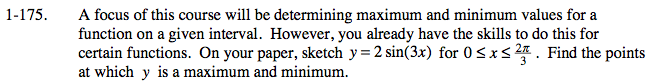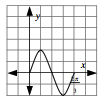### Home > CALC > Chapter 1 > Lesson 1.4.3 > Problem1-175

1-175.

A focus of this course will be determining maximum and minimum values for a function on a given interval. However, you already have the skills to do this for certain functions. On your paper, sketch y = 2 sin(3x) for. Find the points at which y is a maximum and minimum. Homework Help ✎See graph at right.

$\text{It is a sine curve with amplitude 2 and period }\frac{2\pi}{3}.$

The graph is a sine curve that is vertically stretched by a factor of 2 and horizontally compressed by a factor of 3.$\text{Maximum at } \left(\frac{\pi}{6},?\right), \text{ minimum at } (?,-2).$